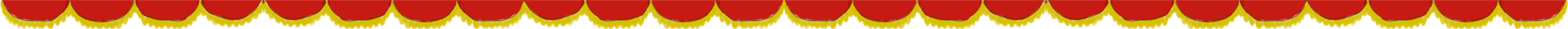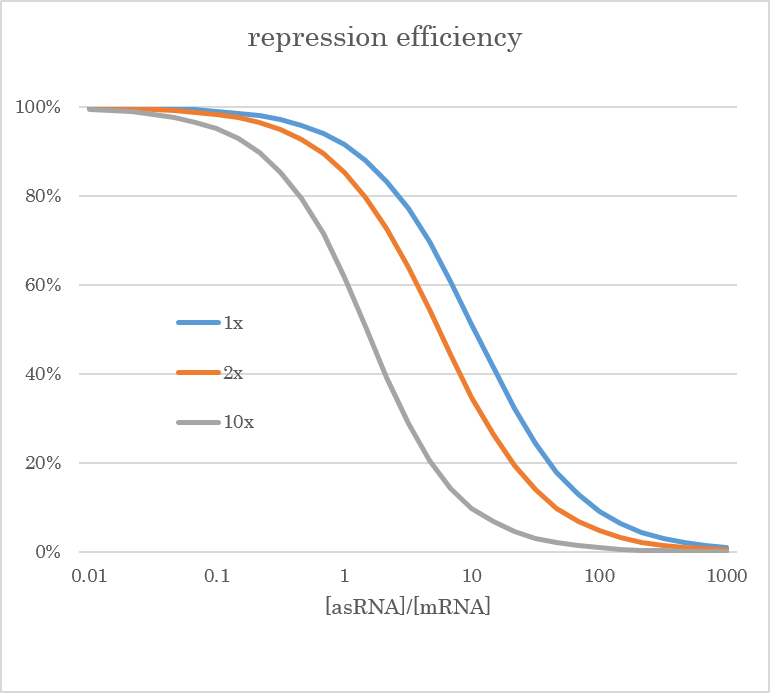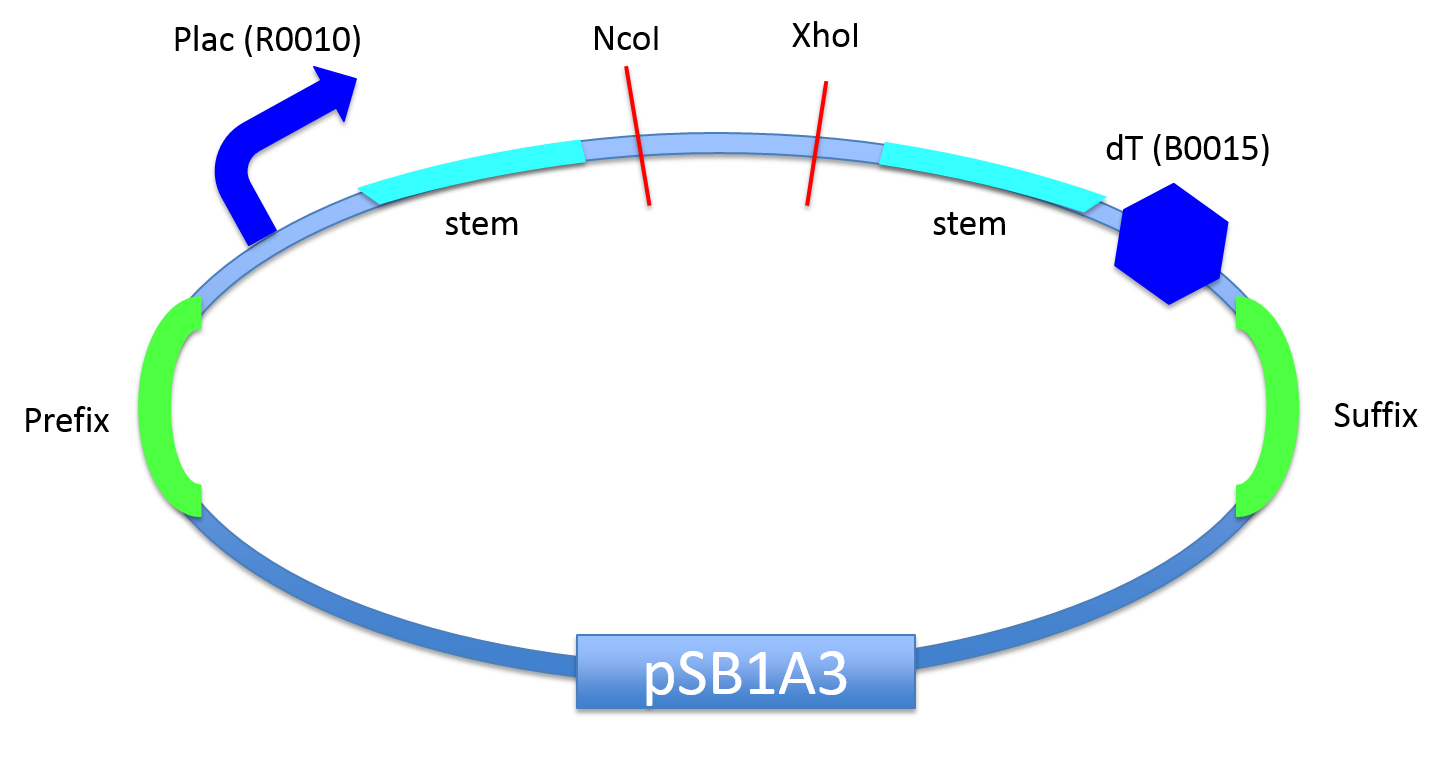# Team:HokkaidoU Japan/Projects/H StemProjects
H-stem System• Top

# Overview

The weak point of silencing genes by anti-sense RNA (asRNA) is the instability of RNA. RNA has a shorter half-life than DNA, so it is essential for asRNA to be stabilized to repress mRNA sufficiently. This problem was solved by inserting each-complementary sequeces called stem regions into upstream and downstream of as RNA (N. Nakashima et al., 2006). These regions make asRNA form stem-loop structures, which lead to increase the stability of asRNA dramatically.

We followed in their footsteps. We designed our original stem sequence "H-stem (BBa_K1524100)" and produced a BioBricked vector for asRNA expression so that other iGEMers and researchers can easily use stemmed asRNA. By using this vector, you can get an asRNA-expressing construct just by inserting PCRed target gene into the vector.

# asRNA stabilization by stem structure

The asRNA region is located between two stem regions. (Fig. 1)Fig. 1 stem and anti-sense region on DNA

Transcripted to RNA, stem sequence binds with each other and make the whole RNA form stem-loop structure. (Fig. 2)Fig. 2 stem and anti-sense region on RNA

This stem-loop structure contributes to stabilize RNA on the following two points.

One is the protection from the exonuclease activity of RNase. Since RNase in the cell decomposes single-strand RNA, stemmed asRNA is hardly affected by such a degradation.

The other is an increase of thermodynamical stability by a decrease of Gibbs energy. Stem region produces such a great amount of negative $\Delta G$ that positive $\Delta G$ produced by the curvature of loop region is overwhelmed.

In bacterial cells, the decomposability of asRNA to that of target mRNA affects the amount of translated mRNA as shown below. Here, x-axis indicates the transcription level of asRNA to that of mRNA.From that graph, you can see that the stability of asRNA greatly contributes to the repression of mRNA. The detail is discussed below.

## Modelling

The reaction of asRNA (hereinafter referred to as $X$), mRNA ($Y$) and RNA duplex ($Z$) consisting of the asRNA and the mRNA in a bacterial cell is

$X+Y \overset{k_{\rm bind}}{\underset{k_{\rm unbind}}{\rightleftharpoons}} Z$

Besides, mRNA and asRNA are produced and decomposed at all times. So, the ordinary differential equation that represses the whole reaction in the cell is shown below.

\begin{cases} \dot{x}=a-bx-k_{\rm bind}xy+k_{\rm unbind}z & \\ \dot{y}=1-y-k_{\rm bind}xy+k_{\rm unbind}z & \\ \dot{z}=k_{\rm bind}xy-k_{\rm unbind}z-cz & \end{cases}

Here, we took $1$ for two constants of the right side first and second terms in the second formula using the flexibilities of $[time]$ and $[concentration]$. Thus, constant $a$ stands for the expression level of asRNA to that of target mRNA and constant $b$ the decomposability of asRNA to that of mRNA.

The unique stable fixed point (in quadrant I) of the ODE is easily found by taking $0$ for each equation. Especially, $y$ value of the fixed point, $y^*$, is

$y=\frac{1}{2} \biggl\{ \sqrt{ \bigl( a-1+\frac{b}{\gamma} \bigl)^2 +4 \frac{b}{\gamma}} - \Bigl( a-1+\frac{b}{\gamma} \Bigl) \biggl\}$

Provided that $\gamma = \frac{k_{\rm bind}c}{k_{\rm unbind}+c}$

We find the graph above by regarding this formula as a function of $a$.

Though here we let $\gamma$ value be $1$ arbitarily, this value does not affect qualitatively as the formula shows.

# Construct

We constructed a vector "H-stem vector (BBa_K1524100)" which includes the H-stem system.Fig. 3 The structure of H-stem system

Stemmed asRNA are translated by a inducible promoter, Plac (BBa_R0010), and it is activated by IPTG induction.

Since anti-sense region can be digested out by NcoI and XhoI, you can insert any anti-sense sequence as recounted above. Additionally, a dummy anti-sense sequence is in advance inserted into this vector.

A point to notice when using our H-stem is that, the stem sequence is difficult to PCR, especially in sequencing PCR. It is supposed that single-strand DNA of this sequence also forms stem-loop structure and it prevents from the extension of complementary strand, but the detailed mechanism is unknown. Therefore, if you want to sequence the H-stem, you have to divide it’s sequence into upstream part and downstream part before doing PCR.

# How to Use

Now, we explain how to use H-stem vector.

What you need is two restriction enzymes, NcoI and XhoI, and two PCR primers, added to the H-stem vector.

1. Determine the asRNA binding sequence, which is about 60 bases, from the tail of RBS to the head of CDS. (Please confirm that the sequence does not include NcoI or XhoI site.)
2. Design the primers which amplify the sequence. Here, add XhoI recognition site (5’-GGGCTCGAG…) to 5’ end of the forward primer and NcoI recognition site (5’-GGGCCATGG…) to that of forward primer.
3. PCR the sequence by the two primers.
4. Digest the PCR product and H-stem vector by NcoI and XhoI, mix them, and ligate together.

We hope that this method will be a help to many iGEMers.

### Reference

1. N. Nakashima et al. (2006) Paired termini stabilize antisense RNAs and enhance conditional gene silencing in Escherichia coli. Nucleic Acids Res 34: 20 e138